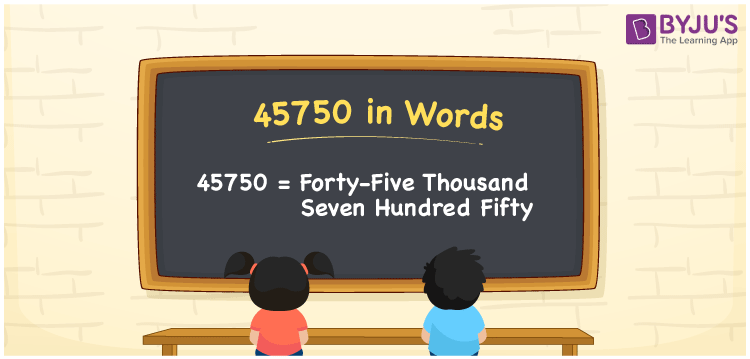# 45750 in words

45750 in words is written as Forty Five Thousand Seven Hundred and Fifty. In 45750, 4 has a place value of ten thousand, 5 has the place value of thousand, 7 is in the place value of hundred and 5 is in the place value of ten. The article on Place Value gives more information. The number 45750 is used in expressions that relate to money, distance, social media views, and many more. For example, “The first installment school fee was Forty Five Thousand Seven Hundred and Fifty rupees”.

 45750 in words Forty Five Thousand Seven Hundred and Fifty Forty Five Thousand Seven Hundred and Fifty in Numbers 45750

## 45750 in English Words## How to Write 45750 in Words?

We can convert 45750 to words using a place value chart. The number 45750 has 5 digits, so let’s make a chart that shows the place value up to 5 digits.

 Ten thousand Thousands Hundreds Tens Ones 4 5 7 5 0

Thus, we can write the expanded form as:

4 × Ten thousand + 5 × Thousand + 7 × Hundred + 5 × Ten + 0 × One

= 4 × 10000 + 5 × 1000 + 7 × 100 + 5 × 10 + 0 × 1

= 45750.

= Forty Five Thousand Seven Hundred and Fifty.

45750 is the natural number that is succeeded by 45749 and preceded by 45751.

45750 in words – Forty Five Thousand Seven Hundred and Fifty.

Is 45750 an odd number? – No.

Is 45750 an even number? – Yes.

Is 45750 a perfect square number? – No.

Is 45750 a perfect cube number? – No.

Is 45750 a prime number? – No.

Is 45750 a composite number? – Yes.

## Solved Example

1. Write the number 45750 in expanded form

Solution: 4 x 10000 + 5 x 1000 + 7 x 100 + 5 x 10 + 0 x 1

We can write 45750 = 40000 + 5000 + 700 + 50 + 0

= 4 x 10000 + 5 x 1000 + 7 x 100 + 5 x 10 + 0 x 1.

## Frequently Asked Questions on 45750 in words

Q1

### How to write the number 45750 in words?

45750 in words is written as Forty Five Thousand Seven Hundred and Fifty.
Q2

### Is 45750 a prime number?

No. 45750 is not a prime number.
Q3

### Is 45750 divisible by 10?

Yes. 45750 is divisible by 10.